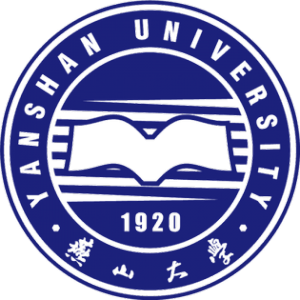﻿ ADVERTS Yanshan University / HEDClub# Yanshan University

Educational events

Yanshan University

France, Bourges

This offer is only for Physical Sciences fields

Yanshan University invites you to participate in the 2019 International Conference on Fractional Calculus Theory and Applications (ICFCTA 2019) that will be held in Bourges, France, on April 25 - 30, 2019. The conference is co-sponsored by LE STUDIUM Loire Valley Institute for Advanced Studies (France), National Institute of Applied Sciences of Centre Val de Loire (France) and Yanshan University (China). This conference aims to provide a platform for researchers in fractional calculus theory and applications to exchange results and advanced technology. During the conference, experts and scholars in related fields and chief editors of well-known magazines will make special reports.
THEMES
Fractional Differential Theory and Application
Fractional Differential Equation Numerical Solution and Application
Variable Fractional Differential Equations Numerical Solution and Applications
Fractional Linear System
Fractional Chaotic System
Fractional Delay Differential Equations Numerical Solution and Applications
Fractional Heat Transfer Model Solution and Application
Large-scale Fractional Calculus Equation Numerical Algorithm Design and Application
Fractional Integral Theory and Application
Fractional Integral Equation Numerical solution and Application
Variable Fractional Integral Equations Numerical Solution and Applications
Fractional Nonlinear System
Fractional Control System
Fractional Delay Differential Equations Numerical Solution and Applications
Local Fractional Calculus Theory and Application
Other Fractional Correlation Theory and Applications
Detailed information about the conference is available at http://www.lestudium-ias.com/fr/event/2019-international-conference-fractional-calculus-theory-and-applications-icfcta-2019

Educational events

Yanshan University

France, Bourges

This offer is only for Physical Sciences fields

Yanshan University invites you to participate in the 2019 International Conference on Fractional Calculus Theory and Applications (ICFCTA 2019) that will be held in Bourges, France, on April 25 - 30, 2019. The conference is co-sponsored by LE STUDIUM Loire Valley Institute for Advanced Studies (France), National Institute of Applied Sciences of Centre Val de Loire (France) and Yanshan University (China). This conference aims to provide a platform for researchers in fractional calculus theory and applications to exchange results and advanced technology. During the conference, experts and scholars in related fields and chief editors of well-known magazines will make special reports. 2019 International Conference on Fractional Calculus Theory and Applications (ICFCTA 2019) includes themes are as follows (but call for papers is not limited to the following areas). THEMES Fractional Differential Theory and Application Fractional Differential Equation Numerical Solution and Application Variable Fractional Differential Equations Numerical Solution and Applications Fractional Linear System Fractional Chaotic System Fractional Delay Differential Equations Numerical Solution and Applications Fractional Heat Transfer Model Solution and Application Large-scale Fractional Calculus Equation Numerical Algorithm Design and Application Fractional Integral Theory and Application Fractional Integral Equation Numerical solution and Application Variable Fractional Integral Equations Numerical Solution and Applications Fractional Nonlinear System Fractional Control System Fractional Delay Differential Equations Numerical Solution and Applications Local Fractional Calculus Theory and Application Other Fractional Correlation Theory and Applications Detailed information about the conference is available at http://www.lestudium-ias.com/fr/event/2019-international-conference-fractional-calculus-theory-and-applications-icfcta-2019